Courses

# Production & Engineering Materials - 2

## 20 Questions MCQ Test GATE Mechanical (ME) 2022 Mock Test Series | Production & Engineering Materials - 2

Description
This mock test of Production & Engineering Materials - 2 for GATE helps you for every GATE entrance exam. This contains 20 Multiple Choice Questions for GATE Production & Engineering Materials - 2 (mcq) to study with solutions a complete question bank. The solved questions answers in this Production & Engineering Materials - 2 quiz give you a good mix of easy questions and tough questions. GATE students definitely take this Production & Engineering Materials - 2 exercise for a better result in the exam. You can find other Production & Engineering Materials - 2 extra questions, long questions & short questions for GATE on EduRev as well by searching above.
QUESTION: 1

Solution:
QUESTION: 2

Solution:
QUESTION: 3

### True centrifugal casting

Solution:
QUESTION: 4

Blind risers

Solution:
QUESTION: 5

In the measurement of surface roughness, heights of successive 10 peaks and troughs were measured from a datum and were 33, 25, 30, 19, 22, 18, 32, 27, 29, and 20 microns. If these measurements were made over a 5 mm length. The CLA value for the given surface is

Solution: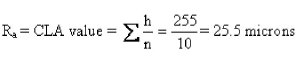*Answer can only contain numeric values
QUESTION: 6

A casting of cube of side 30 cm requires cylindrical side riser. The minimum height of side riser can be chosen is __________ (in mm)

Solution:

Side Riser : For optimum side riser exposed surface area (A) should be minimum,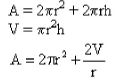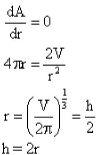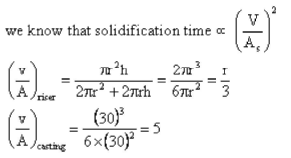at least riser should solidify along with casting. So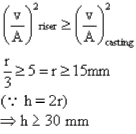QUESTION: 7

The thickness of metallic sheet is reduced from an initial thickness of 16 mm to a final required thickness in one single pass rolling with a pair of cylindrical rollers each of diameter of 400 mm and angle of bits 9.8°. The final required thickness is

Solution:

Given,
Initial thickness (h1) = 16 mm
Final thickness (h2)
Roll diameter = 400 mm
We know that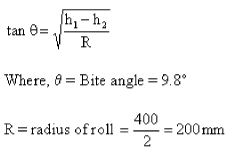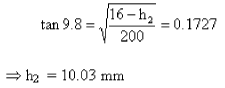*Answer can only contain numeric values
QUESTION: 8

For the given casting of size 40x40x20cm having cylindrical riser of radius 15 cm and height 20cm the time (in sec) required to fill the entire casting and riser is _____

Solution: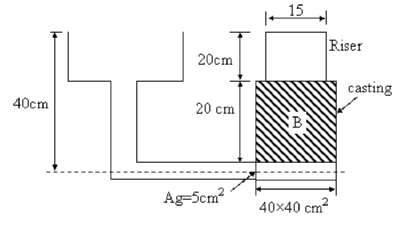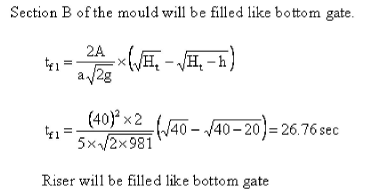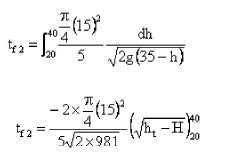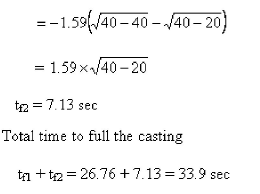QUESTION: 9

The results of machining steel with two grades of tool are given below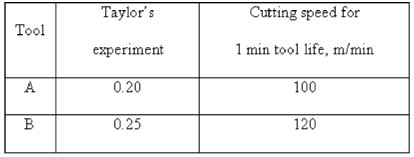For a 180 min tool life which tool is required

Solution: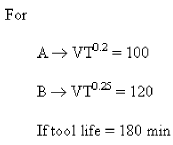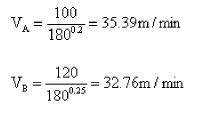Tool A : as VA > VB ⇒ higher speed leads to higher productivity

*Answer can only contain numeric values
QUESTION: 10

An Aluminium rod is drawn into wire of required diameter. Semi die angle is 10°. The friction to be 0.04 and nominal stress 35 MPa. The maximum reduction that can be given to material i s ______________ % .

Solution: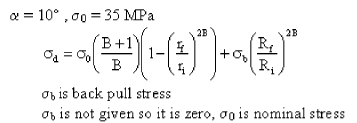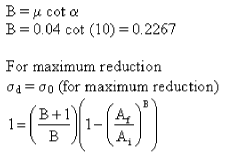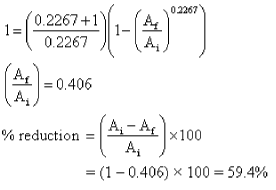QUESTION: 11

A resistance strain gauge experiences 1% change in resistance when subjected to a uniaxial strain of 5000 μm/m. if gauge lactor is assumed as 1.9 then measured value will be

Solution: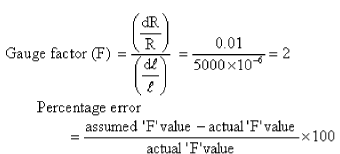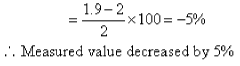*Answer can only contain numeric values
QUESTION: 12

A tube 30mm outer diameter is turned on lathe the cutting velocity of tool is 10 m/min. If rake angle is 35°, depth of cut is 0.15mm, length of chip is 60 mm. The velocity of chip relative to workpiece is _______________(m/min)

Solution: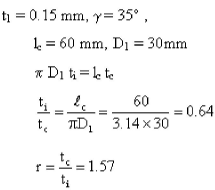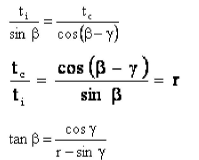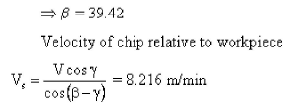QUESTION: 13

A 63.5 mm dia plain milling cutter having 6 tooth is used to face mill a block of aluminum 18cm long and 3 cm wide. The spindle speed is I500 rpm and teed is 0.0125mm/rev, the time taken per cut is

Solution: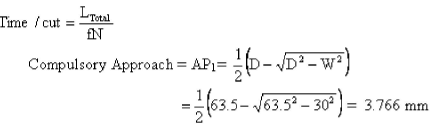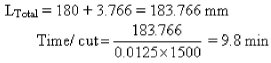*Answer can only contain numeric values
QUESTION: 14

A stepper motor drive having step angle 7.2°/step is used for driving an axis in the NC machine. The motor is connected to the lead screw through a gear having gear ratio (output speed / input speed) is 1/3 and lead screw with pitch of 3 mm & no. of starts is 2. The positional accuracy of the axis of the machine i s _______ μ m.

Solution: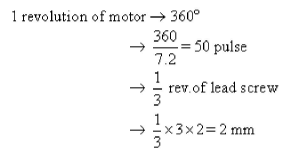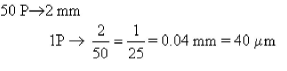QUESTION: 15

A shaft of dimension 40 ± 0.1mm is produced with a circularity error of 0.05mm on maximum material limit. To produce clearance fit between the hole and shaft, the minimum size of the hole required is

Solution:

Mm size of Hole = L-limit of hole
= Maximum Material Limit (MML) of hole
= MML of shaft +circularity error
= 40.1 +0.05 =40.15 mm

*Answer can only contain numeric values
QUESTION: 16

During electric discharge machining operation for drilling a square hole of 10 mm in low carbon steel plate of 5 mm thick, brass tool and kerosene are used. The resistance and capacitance of relaxation circuit are 50 Ω and 10 μF, respectively. The gap is maintained at such a value that the discharge takes place at 150 Volts. If the cycle time of the power supply is 0.7 milliseconds, the average power input in kW for the EDM process will be _____

Solution: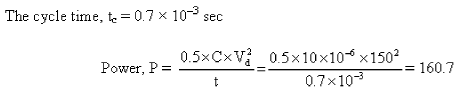W = 0.1607 kW

QUESTION: 17

A shaft of dimension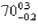is to be inspected by using GO and NOGO gauges. The lower and upper limits of GO gauge respectively when the gauge tolerance and wear allowance each is taken as 10% of work tolerance are

Solution: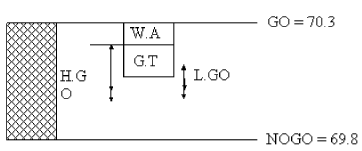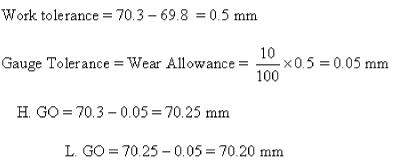QUESTION: 18

The following statements are corresponding to metal forming process

1. Deforming Tungsten at 1000°C is considered as cold working process
2. If the type of load applied is tensile load, it is necessary to maintain load for some more time
3. If the type of load applied is compression load, it is necessary to maintain load for some more time
4. In all the metals increase in temperature causes decrease in yield strength of metal.
5. In case of lead and tin, the cold working is done at temperature below 0°C

Which of the above statement are correct

Solution:

In case compressive type load application it is not necessary to maintain tlie load applied because when the stress induced is greater than the flow stress no elastic deformation is present in the material. In all the metals increase in temperature causes reduction in yield stress except steel.

QUESTION: 19

An L-shaped component is produced by bending with total leg length of horizontal leg is 80mm and vertical leg is 60mm and inside radius of the corner is 8mm and thickness of the sheet is 2mm. The developed length of the strip is

Solution: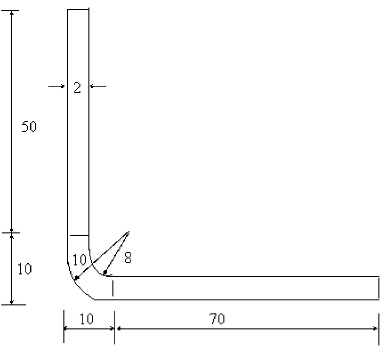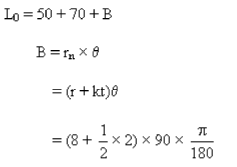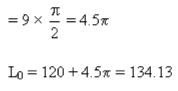QUESTION: 20

A 24 teeth gear is to be produced using simple indexing mechanism, the following plates can be used

Plate 1: 15, 16, 17, 18, 19 and 20
Plate 2: 21, 23, 27, 29, 31 and 33
Plate 3: 35, 37, 39,41,43,47 and 49

Solution:

N = 24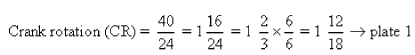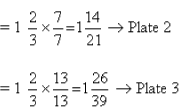Hence all the 3 - plates can be used.